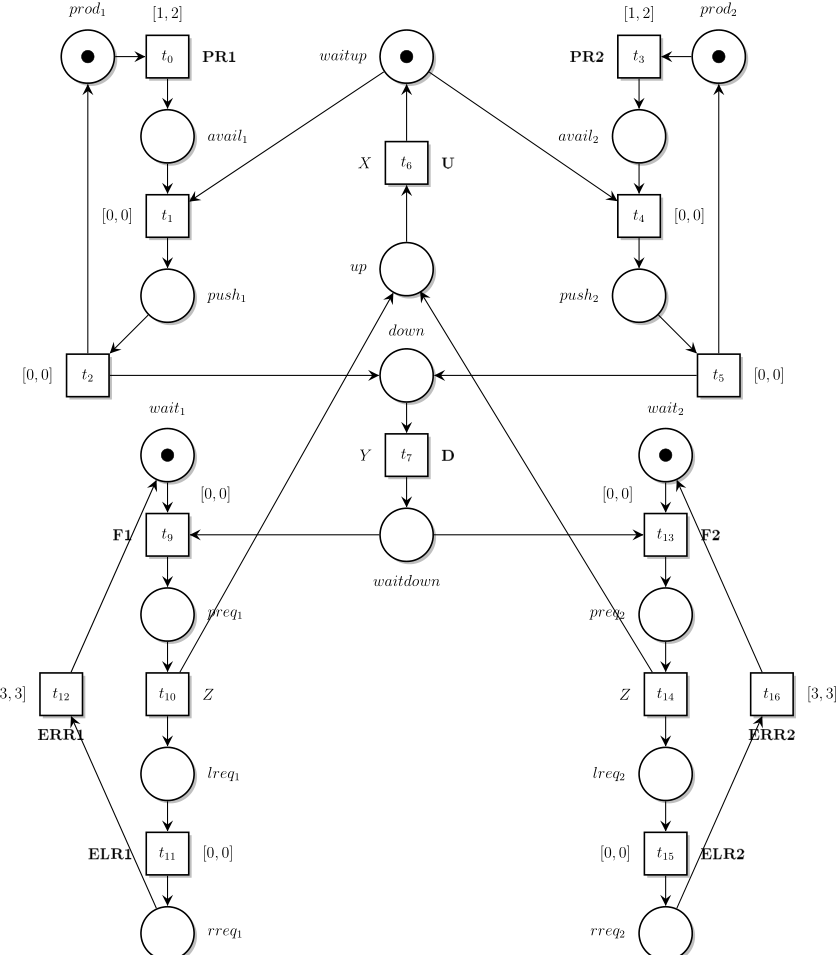# Reproducing the Benchmarks of our 2020 Paper

This post is part II of a series of articles describing how to reproduce the experiments reported in our 2020 (submitted) paper about Twina. See part I.

## Pattern Diagnosability on the Product Transportation System

In this post we consider the model of a product transportation system with added timing constraints displayed in Fig. 1.Model for the product transfer system with parametric time intervals on t6, t7, t10 and t14 (drawn from transport_timed.net using nd)

As in part I, we consider the diagnosability with respect to omega , a pattern for property “at least three F1 before a F2”. Hence the observable labels in this example are U, D, PR1, PR2, ERR1, ERR2, ELR1 and ELR2.

It is possible to check the diagnosability of the pattern using a sequence of bash commands such as:

$cat transport.tpn load transport_timed.net load omega.net intersect dup 1 intersect U D PR1 PR2 ERR1 ERR2 ELR1 ELR2$ twina -kaut transport.tpn | ktzio -tina -AUT - transport.ktz
$selt -q -b transport.ktz -f "(<> {found.1.1}) => <>({found.1.2} \/ dead);" FALSE  We have tested the diagnosability of the system for several choices of timing constraints for the transitions t6 (label U, denoting a product going Up the elevator), t7 (label D, denoting a product going Down the elevator), t10 and t14 (silent, non-observable transitions.). The result depends on the timing constraints: let X be the (static) time interval for t6, Y for t7 and Z for t10 and t14. •$X = Y = Z = [0, \infty[$: the system is not diagnosable and has 126 548 classes. The system without any timing constraint leads to a state space with 14 270 markings •$X = Y = [0, \infty[$and$Z = [0, 6]$: the system is not diagnosable and has 129 096 classes •$X = Y = [0, \infty[$and$Z = [1, 6]$: the system is diagnosable and has 15 848 classes •$X = Y = [1, 10]$and$Z = [0, \infty[\$: the system is diagnosable and has only 2186 classes

The time needed to generate the state space in the most complex case is about 4.5s on a laptop (see model transport_bigger ), and under 10ms for the models with less than 20 000 classes.